Updating search results...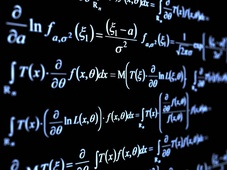# Approaching Mathematics with Open Access Common Core Courses

25 affiliated resources

# Search Resources

View
Selected filters: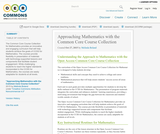Conditional Remix & Share Permitted
CC BY-NC
Rating
0.0 stars

The Common Core Course Collection for Mathematics provides an innovative and engaging curriculum that will help students achieve the goals of CCSS for Mathematics. The courses provide flexibility in presentation of
materials with technology-supported lessons and components that facilitate student engagement. While challenging students to reach the higher standards incorporated in the CCSS for Mathematics, the courses are easily adaptable for students at all levels.

Subject:
Mathematics
Material Type:
Teaching/Learning Strategy
Author:
Melinda Boland
01/28/2016Only Sharing Permitted
CC BY-NC-ND
Rating
4.0 stars

This lesson unit is intended to help teachers assess how well students are able to classify solutions to a pair of linear equations by considering their graphical representations. In particular, this unit aims to help teachers identify and assist students who have difficulties in: using substitution to complete a table of values for a linear equation; identifying a linear equation from a given table of values; and graphing and solving linear equations.

Subject:
Algebra
Material Type:
Assessment
Lesson Plan
Provider:
Shell Center for Mathematical Education
Provider Set:
Mathematics Assessment Project (MAP)
04/26/2013Only Sharing Permitted
CC BY-NC-ND
Rating
0.0 stars

This lesson unit is intended to help teachers assess how well students are able to interpret exponential and linear functions and in particular to identify and help students who have the following difficulties: translating between descriptive, algebraic and tabular data, and graphical representation of the functions; recognizing how, and why, a quantity changes per unit intervale; and to achieve these goals students work on simple and compound interest problems.

Subject:
Algebra
Measurement and Data
Material Type:
Assessment
Lesson Plan
Provider:
Shell Center for Mathematical Education
Provider Set:
Mathematics Assessment Project (MAP)
04/26/2013Only Sharing Permitted
CC BY-NC-ND
Rating
3.0 stars

This lesson unit is intended to help teachers assess how well students are able to: use the Pythagorean theorem to derive the equation of a circle; and translate between the geometric features of circles and their equations.

Subject:
Geometry
Material Type:
Assessment
Lesson Plan
Provider:
Shell Center for Mathematical Education
Provider Set:
Mathematics Assessment Project (MAP)
04/26/2013Only Sharing Permitted
CC BY-NC-ND
Rating
0.0 stars

This lesson unit is intended to help teachers assess how well students are able to: translate between the equations of circles and their geometric features; and sketch a circle from its equation.

Subject:
Geometry
Material Type:
Assessment
Lesson Plan
Provider:
Shell Center for Mathematical Education
Provider Set:
Mathematics Assessment Project (MAP)
04/26/2013Only Sharing Permitted
CC BY-NC-ND
Rating
4.5 stars

This lesson unit is intended to help you assess how well students are able to: solve simple problems involving ratio and direct proportion; choose an appropriate sampling method; and collect discrete data and record them using a frequency table.

Subject:
Education
Geometry
Measurement and Data
Ratios and Proportions
Material Type:
Assessment
Lecture Notes
Lesson Plan
Teaching/Learning Strategy
Provider:
Shell Center for Mathematical Education
Provider Set:
Mathematics Assessment Project (MAP)
Author:
http://map.mathshell.org/
04/26/2013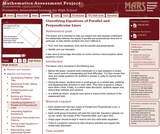Only Sharing Permitted
CC BY-NC-ND
Rating
0.0 stars

This lesson unit is intended to help teachers assess how well students are able to understand the relationship between the slopes of parallel and perpendicular lines and, in particular, to help identify students who find it difficult to: find, from their equations, lines that are parallel and perpendicular; and identify and use intercepts. It also aims to encourage discussion on some common misconceptions about equations of lines.

Subject:
Mathematics
Material Type:
Assessment
Lesson Plan
Provider:
Shell Center for Mathematical Education
Provider Set:
Mathematics Assessment Project (MAP)
04/26/2013Only Sharing Permitted
CC BY-NC-ND
Rating
3.0 stars

This lesson unit is intended to help you assess how well students working with square numbers are able to: choose an appropriate, systematic way to collect and organize data, examining the data for patterns; describe and explain findings clearly and effectively; generalize using numerical, geometrical, graphical and/or algebraic structure; and explain why certain results are possible/impossible, moving towards a proof.

Subject:
Algebra
Geometry
Measurement and Data
Material Type:
Assessment
Lesson Plan
Provider:
Shell Center for Mathematical Education
Provider Set:
Mathematics Assessment Project (MAP)
04/26/2013Only Sharing Permitted
CC BY-NC-ND
Rating
0.0 stars

This lesson unit is intended to help teachers assess how well students are able to use geometric properties to solve problems. In particular, the lesson will help you identify and help students who have the following difficulties: solving problems by determining the lengths of the sides in right triangles; and finding the measurements of shapes by decomposing complex shapes into simpler ones. The lesson unit will also help students to recognize that there may be different approaches to geometrical problems, and to understand the relative strengths and weaknesses of those approaches.

Subject:
Geometry
Material Type:
Assessment
Lesson Plan
Provider:
Shell Center for Mathematical Education
Provider Set:
Mathematics Assessment Project (MAP)
04/26/2013Only Sharing Permitted
CC BY-NC-ND
Rating
0.0 stars

This lesson unit is intended to help teachers assess how well students are able to use geometric properties to solve problems. In particular, it will help you identify and help students who have difficulty: decomposing complex shapes into simpler ones in order to solve a problem; bringing together several geometric concepts to solve a problem; and finding the relationship between radii of inscribed and circumscribed circles of right triangles.

Subject:
Geometry
Material Type:
Assessment
Lesson Plan
Provider:
Shell Center for Mathematical Education
Provider Set:
Mathematics Assessment Project (MAP)
04/26/2013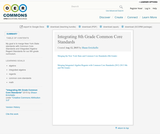Unrestricted Use
CC BY
Rating
0.0 stars

My goal is to merge New York State standards with Common Core Standards and Integrated Algebra Regent Standards for our 8th grade curriculum.

Subject:
Mathematics
Material Type:
Full Course
Lesson Plan
Syllabus
Author:
Shaun Errichiello
01/28/2016Only Sharing Permitted
CC BY-NC-ND
Rating
4.0 stars

This lesson unit is intended to help you assess how well students are able to: Perform arithmetic operations, including those involving whole-number exponents, recognizing and applying the conventional order of operations; Write and evaluate numerical expressions from diagrammatic representations and be able to identify equivalent expressions; apply the distributive and commutative properties appropriately; and use the method for finding areas of compound rectangles.

Subject:
Geometry
Material Type:
Assessment
Lesson Plan
Provider:
Shell Center for Mathematical Education
Provider Set:
Mathematics Assessment Project (MAP)
04/26/2013Conditional Remix & Share Permitted
CC BY-NC
Rating
0.0 stars

Four full-year digital course, built from the ground up and fully-aligned to the Common Core State Standards, for 7th grade Mathematics. Created using research-based approaches to teaching and learning, the Open Access Common Core Course for Mathematics is designed with student-centered learning in mind, including activities for students to develop valuable 21st century skills and academic mindset.

Subject:
Mathematics
Material Type:
Full Course
Provider:
Pearson
10/06/2016Rating
0.0 stars

Algebraic Reasoning

Type of Unit: Concept

Prior Knowledge

Students should be able to:

Add, subtract, multiply, and divide rational numbers.
Evaluate expressions for a value of a variable.
Use the distributive property to generate equivalent expressions including combining like terms.
Understand solving an equation or inequality as a process of answering a question: which values from a specified set, if any, make the equation or inequality true?
Write and solve equations of the form x+p=q and px=q for cases in which p, q, and x are non-negative rational numbers.
Understand and graph solutions to inequalities x<c or x>c.
Use equations, tables, and graphs to represent the relationship between two variables.
Relate fractions, decimals, and percents.
Solve percent problems included those involving percent of increase or percent of decrease.

Lesson Flow

This unit covers all of the Common Core State Standards for Expressions and Equations in Grade 7. Students extend what they learned in Grade 6 about evaluating expressions and using properties to write equivalent expressions. They write, evaluate, and simplify expressions that now contain both positive and negative rational numbers. They write algebraic expressions for problem situations and discuss how different equivalent expressions can be used to represent different ways of solving the same problem. They make connections between various forms of rational numbers. Students apply what they learned in Grade 6 about solving equations such as x+2=6 or 3x=12 to solving equations such as 3x+6=12 and 3(x−2)=12. Students solve these equations using formal algebraic methods. The numbers in these equations can now be rational numbers. They use estimation and mental math to estimate solutions. They learn how solving linear inequalities differs from solving linear equations and then they solve and graph linear inequalities such as −3x+4<12. Students use inequalities to solve real-world problems, solving the problem first by arithmetic and then by writing and solving an inequality. They see that the solution of the algebraic inequality may differ from the solution to the problem.

Subject:
Mathematics
Algebra
Material Type:
Unit of Study
Provider:
Pearson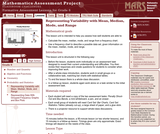Only Sharing Permitted
CC BY-NC-ND
Rating
0.0 stars

This lesson unit is intended to help you assess how well students are able to: Calculate the mean, median, mode, and range from a frequency chart; and to use a frequency chart to describe a possible data set, given information on the mean, median, mode, and range.

Subject:
Education
Mathematics
Physics
Material Type:
Activity/Lab
Assessment
Lesson Plan
Provider:
Shell Center for Mathematical Education
Provider Set:
Mathematics Assessment Project (MAP)
Author:
Shell Center Team
01/17/2013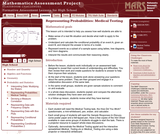Only Sharing Permitted
CC BY-NC-ND
Rating
0.0 stars

This lesson unit is intended to help teachers assess how well students are able to: make sense of a real life situation and decide what math to apply to the problem; understand and calculate the conditional probability of an event A, given an event B, and interpret the answer in terms of a model; represent events as a subset of a sample space using tables, tree diagrams, and Venn diagrams; and interpret the results and communicate their reasoning clearly.

Subject:
Statistics and Probability
Material Type:
Assessment
Lesson Plan
Provider:
Shell Center for Mathematical Education
Provider Set:
Mathematics Assessment Project (MAP)
04/26/2013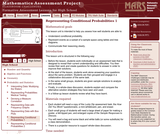Only Sharing Permitted
CC BY-NC-ND
Rating
0.0 stars

This lesson unit is intended to help teachers assess how well students are able to: Understand conditional probability; represent events as a subset of a sample space using tables and tree diagrams; and communicate their reasoning clearly.

Subject:
Statistics and Probability
Material Type:
Assessment
Lesson Plan
Provider:
Shell Center for Mathematical Education
Provider Set:
Mathematics Assessment Project (MAP)
04/26/2013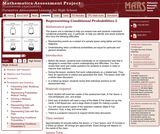Only Sharing Permitted
CC BY-NC-ND
Rating
0.0 stars

This lesson unit is intended to help teachers assess how well students understand conditional probability, and, in particular, to help teachers identify and assist students who have the following difficulties: representing events as a subset of a sample space using tables and tree diagrams; and understanding when conditional probabilities are equal for particular and general situations.

Subject:
Statistics and Probability
Material Type:
Assessment
Lesson Plan
Provider:
Shell Center for Mathematical Education
Provider Set:
Mathematics Assessment Project (MAP)
04/26/2013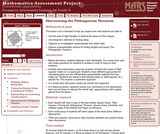Only Sharing Permitted
CC BY-NC-ND
Rating
4.0 stars

This lesson unit is intended to help teachers assess how well students are able to: use the area of right triangles to deduce the areas of other shapes; use dissection methods for finding areas; organize an investigation systematically and collect data; deduce a generalizable method for finding lengths and areas (The Pythagorean Theorem.)

Subject:
Geometry
Material Type:
Assessment
Lesson Plan
Provider:
Shell Center for Mathematical Education
Provider Set:
Mathematics Assessment Project (MAP)
04/26/2013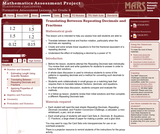Only Sharing Permitted
CC BY-NC-ND
Rating
4.0 stars

This lesson unit is intended to help teachers assess how well students are able to: translate between decimal and fraction notation, particularly when the decimals are repeating; create and solve simple linear equations to find the fractional equivalent of a repeating decimal; and understand the effect of multiplying a decimal by a power of 10.

Subject:
Algebra
Ratios and Proportions
Material Type:
Assessment
Lesson Plan
Provider:
Shell Center for Mathematical Education
Provider Set:
Mathematics Assessment Project (MAP)